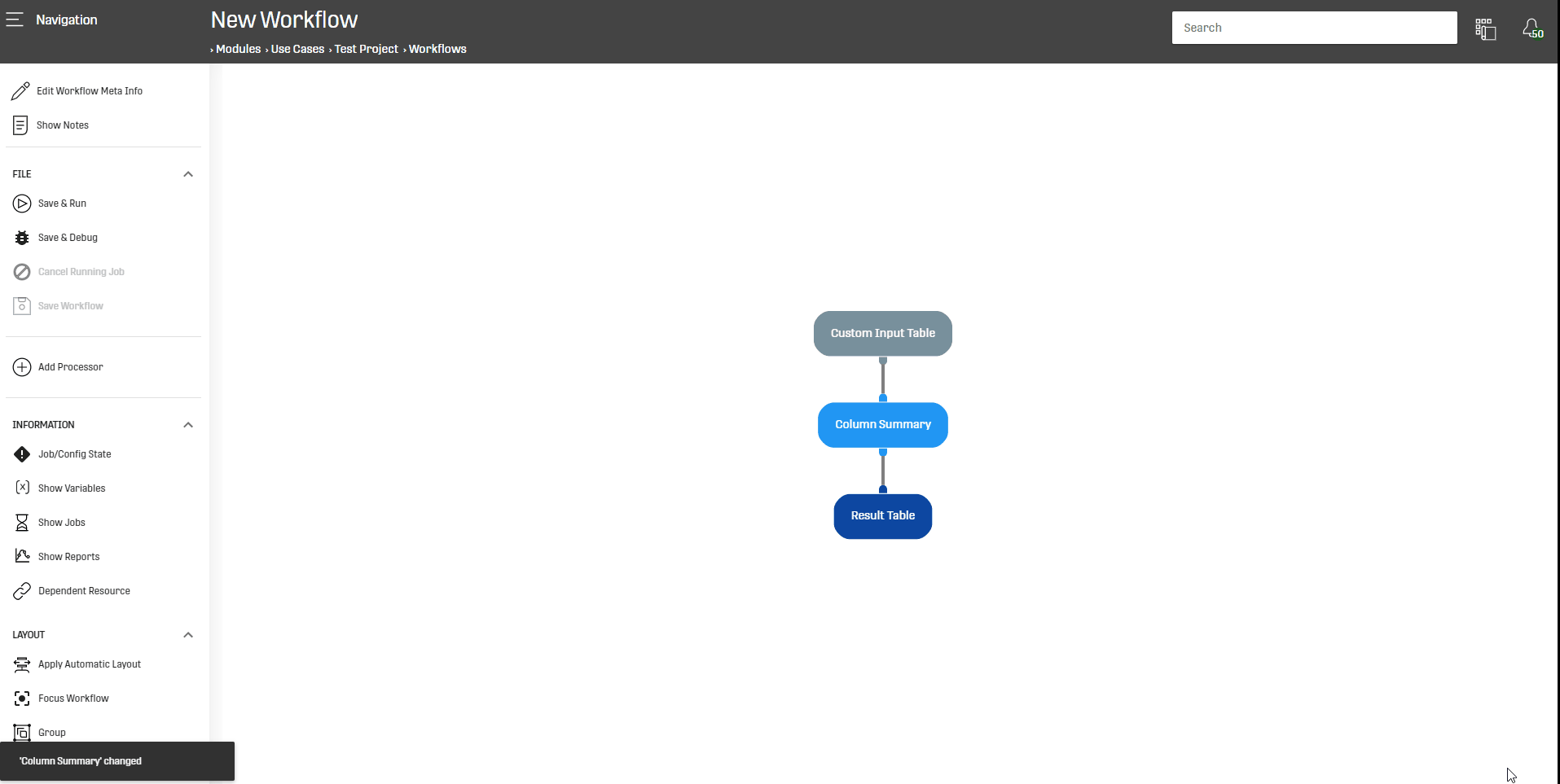# Column Summary Processor

Modified on Tue, 30 Nov 2021 at 11:37 AM

# Overview

This processor provides descriptive summaries (mean, variance, amount of non-zero values, total number of values) for all of the input Data numeric columns.

# Motivation

Workflows in ONE DATA enable the user to exploit and work with large Data, but to be able to extract Value from this data the user must have a general overview of it.

It would be very helpful and time saving to have an effective tool which can present descriptive summaries of the Data, and that is the functionality of this processor.

# Configuration

This processor does not need configuration.

What is important thing is to provide the processor with input Data which contains at least one numerical column.

The data can come from a Dataset Load, a Data Table Load or a Custom Input Table.

# Output

This processor generates short summaries for each numeric variable in the dataset including its column names, mean values, variances, number of non-zero values and the number of rows in the dataset.

So the output looks as follows:

 Column Name:the list of all numerical columns from input data Mean:the mean value of the column of interest Variance:How values vary around the Mean Non Zero:total of non zero values Total:Total number of values

# Example

In this example, the Column Summary Processor will be used on a toy Dataset generated by a Custom Input Table:# Related Articles

Distinct Summary Processor

Heuristic Summaries Processor